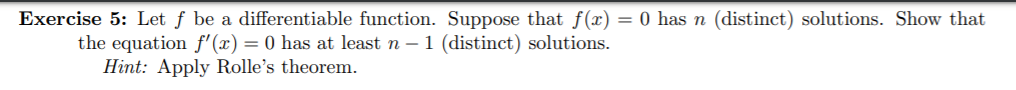# Exercise 5: Let f be a differentiable function. Suppose that f(x) = 0 has n (distinct) solutions. Show thatthe equation f' (x) = 0 has at least n - 1 (distinct) solutionsHint: Apply Rolle's theorem.

Question
18 viewshelp_outlineImage TranscriptioncloseExercise 5: Let f be a differentiable function. Suppose that f(x) = 0 has n (distinct) solutions. Show that the equation f' (x) = 0 has at least n - 1 (distinct) solutions Hint: Apply Rolle's theorem. fullscreen
check_circle

Step 1

Rolle's theorem states that if a function f is continuous on the closed interval [a, b] and differentiable on the open interval (a, b) such that f(a) = f(b), then f′(x) = 0 for some x with a ≤ x ≤ b.

Given f(x)=0 has n distinct solutions. Let, x1 , x2 , x3 , x4 , ...... , xn

Then, f(x1)= f(x2) = f(x3) = f(x4) = .... =f(xn)=0

Step 2

Since, f(x1)=f(x2)=0 so by Rolle's theorem  f′(p1) = 0 for some p1 with x1 ≤ p1 ≤ x2. (There can be more than one point p1)

Since, f(x2)=f(x3)=0 so by Rolle's theorem  f′(p2) = 0 for some p2 with x2 ≤...

### Want to see the full answer?

See Solution

#### Want to see this answer and more?

Solutions are written by subject experts who are available 24/7. Questions are typically answered within 1 hour.*

See Solution
*Response times may vary by subject and question.
Tagged in

### Other